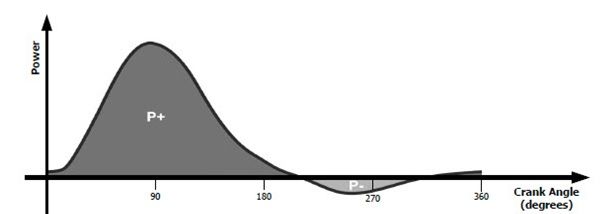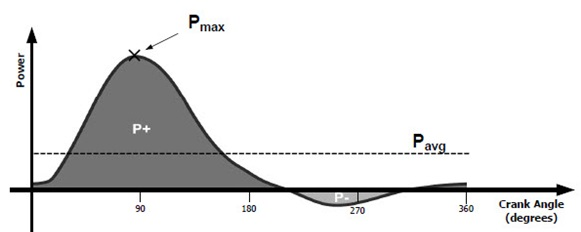# Pedal Smoothness and Torque Effectiveness

## Torque Effectiveness

The Torque Effectiveness (TE) metric is calculated by analyzing the positive (forward) and negative (backward) torque applied to the crank over each revolution (you need the power meter with advanced pedal metrics - Garmin Vector 2, PowerTap P1 etc).

The following diagram shows a typical power curve for one crank arm, where P+ represents the positive power applied to the crank and is the sum of the instantaneous power measurements. Similarly, P- is the sum of the negative instantaneous power measurements. P- is typically produced when the backstroke of one leg is opposing the downstroke of the other leg.Torque Effectiveness = (((P+) + (P-))/(P+)) x 100

## Pedal Smoothness

The Pedal Smoothness (PS) metric is calculated on Power Meter by dividing the average power (Pavg) by the peak power (Pmax) across one crank cycle (see the diagram below). It is a measurement of how evenly power was applied throughout all 360 degrees of a pedal stroke.

The shape of the power curve and the resulting value of Pedal Smoothness will vary depending on the style of pedaling.Pedal Smoothness = (Pavg/Pmax) x 100